CAT  >  IIFT Quantitative Ability MCQ Quiz - 1

# IIFT Quantitative Ability MCQ Quiz - 1

Test Description

## 22 Questions MCQ Test IIFT Mock Test Series | IIFT Quantitative Ability MCQ Quiz - 1

IIFT Quantitative Ability MCQ Quiz - 1 for CAT 2022 is part of IIFT Mock Test Series preparation. The IIFT Quantitative Ability MCQ Quiz - 1 questions and answers have been prepared according to the CAT exam syllabus.The IIFT Quantitative Ability MCQ Quiz - 1 MCQs are made for CAT 2022 Exam. Find important definitions, questions, notes, meanings, examples, exercises, MCQs and online tests for IIFT Quantitative Ability MCQ Quiz - 1 below.
Solutions of IIFT Quantitative Ability MCQ Quiz - 1 questions in English are available as part of our IIFT Mock Test Series for CAT & IIFT Quantitative Ability MCQ Quiz - 1 solutions in Hindi for IIFT Mock Test Series course. Download more important topics, notes, lectures and mock test series for CAT Exam by signing up for free. Attempt IIFT Quantitative Ability MCQ Quiz - 1 | 22 questions in 20 minutes | Mock test for CAT preparation | Free important questions MCQ to study IIFT Mock Test Series for CAT Exam | Download free PDF with solutions
 1 Crore+ students have signed up on EduRev. Have you?
IIFT Quantitative Ability MCQ Quiz - 1 - Question 1

### In the figure below, AB = 20 cm, BC = 13 cm and AC = 11 cm. It is given that the area of ΔABC is 66 sq. cm. What is the area (in sq. cm) of the semicircle?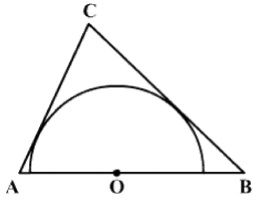Detailed Solution for IIFT Quantitative Ability MCQ Quiz - 1 - Question 1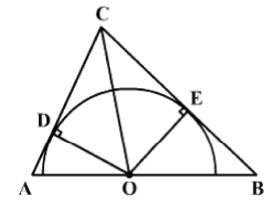Draw perpendiculars from O on to AC and BC. Let them meet AC and BC at D and E respectively. D and E lie on the circumference of the semicircle, since the radius and the tangent at the point of contact are perpendicular. Draw OC.
Let the radius of the semicircle be R.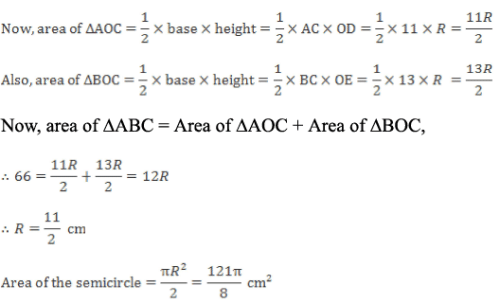Hence, option 3.

IIFT Quantitative Ability MCQ Quiz - 1 - Question 2

### In a 4000 metre race around a circular stadium having circumference 1000 metres, the fastest runner and the slowest runner reach the same point at the end of the 5th minute, for the first time after the start of the race. All the runners start running from the same point and each runner maintains uniform speed throughout the race. If the fastest runner runs at twice the speed of the slowest runner, what is the time taken by the fastest runner to finish the race?

Detailed Solution for IIFT Quantitative Ability MCQ Quiz - 1 - Question 2

The ratio of the speeds of the fastest and the slowest runner is 2 : 1. i.e. They will meet for the first time, when the fastest runner completes 2 rounds while the slowest runner completes 1 round. Now, they meet for the first time after 5 min. The fastest runner completes 2 rounds in 5 min. i.e. he covers 2000 metres in 5 min.
Thus, the fastest runner completes the race i.e. covers 4000 metres in 10 min. Hence option 1.

IIFT Quantitative Ability MCQ Quiz - 1 - Question 3

### A cow is tethered, using a rope of 20 ft, to a comer of a rectangular house which measures 16 ft by 10 ft, situated in the middle of a very big grass land. What is the maximum area that can be grazed by the cow?

Detailed Solution for IIFT Quantitative Ability MCQ Quiz - 1 - Question 3

Refer the diagram,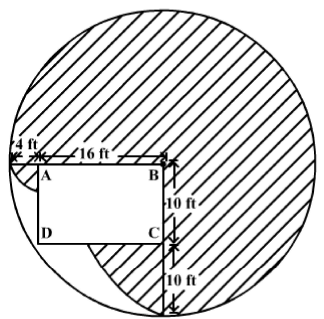The shaded region represents the maximum area that can be grazed by the cow.

Let the cow be tethered to the top right comer (point B) of the rectangular house ABCD.
From the figure, it can be seen that: (i) The cow can graze 3/4th of the area of the circle of radius 20 ft. (ii) Moving to the left, along the length of the house the rope can go up to 16 ft along the boundary of the house. The remaining 4 ft of the rope would enable the cow to graze a small quarter circle of radius 4 ft. (iii) Moving down, along the breath of the house, the rope can go up to 10 ft along the boundary of the house. The remaining 10 ft of the rope would enable the cow to graze another quarter circle of radius 10 ft. The maximum total area that the cow can graze.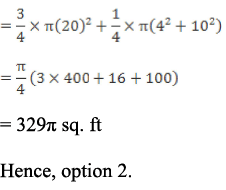IIFT Quantitative Ability MCQ Quiz - 1 - Question 4

What is the arithmetic mean of {xn, xn + 1, ..., x2n}, where x, n > 1?

Detailed Solution for IIFT Quantitative Ability MCQ Quiz - 1 - Question 4

Given series: {xn, xn + 1, . . x2n}.
It can be seen that this series is a geometric progression with the first term = a = xn and the common ratio = r = x
The number of terms of the series = 2n - n + 1 = (n + 1) terms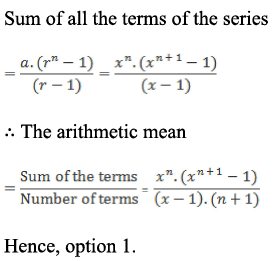IIFT Quantitative Ability MCQ Quiz - 1 - Question 5

Mani invested a sum of Rs. 60,000 with IPO of Accrue Corporation in return for shares of face/issue value of Rs. 10 in the year 2006. In the year 2007, Accrue declared a 2 for 1 stock split together with a bonus share allotment in the ratio 5 : 12. A dividend of 15% was also declared in the year 2007. In the year 2008, the company announced a bonus shares allotment in the ratio 11 : 17 and a dividend of 12%. What is the percentage return for Mani in terms of the dividend obtained? Assume that the dividend is not invested and the value is not affected by inflation.

Detailed Solution for IIFT Quantitative Ability MCQ Quiz - 1 - Question 5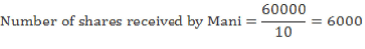Year 2007:
In a stock split, the number of shares increases decreasing the face value of the stock. Since it was a 2 for 1 stock split, Mani’s shares increase to 12000 and the face value of each share reduces to Rs. 5.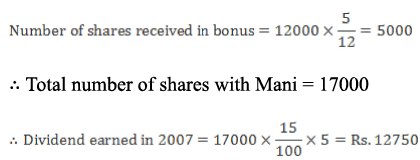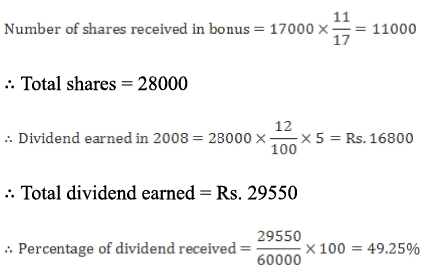Hence, option 2.

IIFT Quantitative Ability MCQ Quiz - 1 - Question 6

In a building on the eve of Holi, the residents put up six identical tanks filled with water colours, each with three outlet hoses. Each hose can individually empty the tank to which it is attached in 20 minutes, 30 minutes and 15 minutes respectively. In order to prevent the colours from getting depleted soon, there is also an overhead tap over each tank that pours in fresh colours. The tap can fill the tank underneath it completely in 10 minutes. How long can the residents play Holi with one tank before it gets depleted completely, assuming that all the three outlet hoses and the overhead tap are working simultaneously and continuously?

Detailed Solution for IIFT Quantitative Ability MCQ Quiz - 1 - Question 6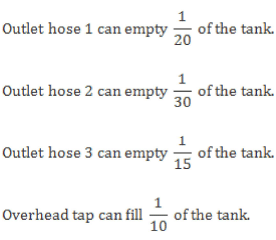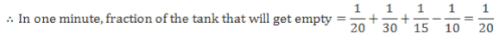It will take a total of 20 minutes for one tank to be depleted.
Hence, option 2.

IIFT Quantitative Ability MCQ Quiz - 1 - Question 7

A bartender pilfers wine from casks. He removes daily 3 litres of Soolah wine from a 30 litres cask of pure soolah wine and replaces it with 2 litres of water and 1 litres of Marcus wine. He continues doing this for a whole week. What is the ratio of soolah wine to water to marcus wine in the cask at the end of the week?

Detailed Solution for IIFT Quantitative Ability MCQ Quiz - 1 - Question 7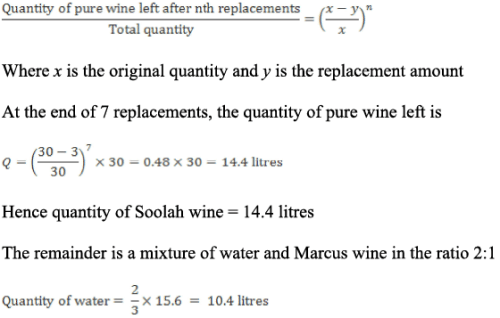Quantity of Marcus wine = 15.6 - 10.4 = 5.2 litres.
Hence the ratio = 14.4 : 10.4 : 5.2 = 36 : 26 : 13. Hence, option 2.

IIFT Quantitative Ability MCQ Quiz - 1 - Question 8

A sheet of paper is cut in the form of an equilateral triangle of side x cm. A long pin is passed through the centroid of the triangle such that it is perpendicular to the paper. If the paper is now spun around the pin, then what is the area of the figure thus formed?

Detailed Solution for IIFT Quantitative Ability MCQ Quiz - 1 - Question 8

The long pin forms the axis of rotation of the given triangle, and since it passes through the centroid; when the triangle is rotated, it rotates along its centroid thus creating a circumcircle around the triangle. The radius of this circumcircle is equal to line joining the centroid to one of the vertex of the triangle. In an equilateral triangle, the altitudes and the medians are the same and the centroid is also the circumcentre. The centroid divides the altitude in the ratio of 2 : 1.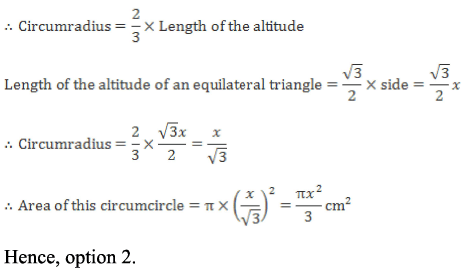IIFT Quantitative Ability MCQ Quiz - 1 - Question 9

A population of 16000 x 1054 bacteria is required to decompose waste in a landfill. The amount of waste in the landfill is 256 x 1027 kg. How many bacteria would be required to decompose 144 x 109 kg of waste?

Detailed Solution for IIFT Quantitative Ability MCQ Quiz - 1 - Question 9

Bacteria required to decompose 256 x 1027 kg of waste = 16000 x 1054.

Bacteria required to decompose 144 x 109 kg of waste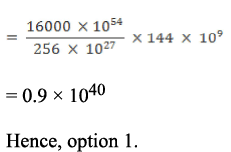IIFT Quantitative Ability MCQ Quiz - 1 - Question 10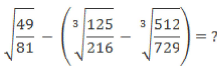Detailed Solution for IIFT Quantitative Ability MCQ Quiz - 1 - Question 10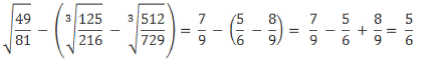Hence, option 1.

IIFT Quantitative Ability MCQ Quiz - 1 - Question 11

Rachel needs a pink hair band. Monica has 2 pink and 3 blue hair bands and Phoebe has 3 pink and 4 blue hair bands. Because they are friends, Monica takes one hair band from Phoebe in exchange for a chocolate muffin. Monica then allows Rachel to pick one hair band from her hair band bag without taking a look at the contents of the bag. What is the probability that Rachel will get what she needs?

Detailed Solution for IIFT Quantitative Ability MCQ Quiz - 1 - Question 11

Let E = Event of Monica taking a Pink hair band from Phoebe E’ = Event of Monica taking a blue hair band from Phoebe B = Event of Rachel picking a Pink hair band from Monica’s hair band bag.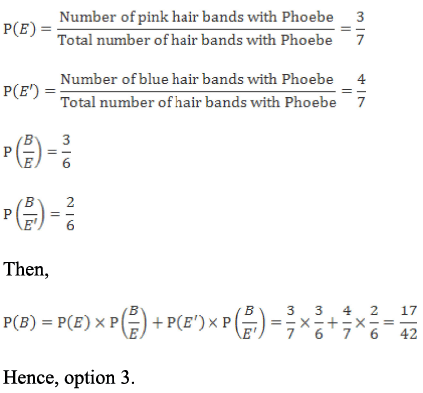IIFT Quantitative Ability MCQ Quiz - 1 - Question 12

Answer the following question based on the information given below.

f(x) = x3 - 2x2 - 5x + 6

g(x) = ln(sin x)

What is the range of g(f(x))?

Detailed Solution for IIFT Quantitative Ability MCQ Quiz - 1 - Question 12

g(f(x )) = ln[sin(x3 - 2x2 - 5x + 6)]

x3 - 2x2 - 5x + 6 = (x - 1)(x + 2)(x - 3)

As the argument of a natural logarithm has to be positive, g(f(x)) cannot defined for all the values of x, for which sin(x3 - 2x2 - 5x + 6) gives a non positive value.
The range of sin x is -1 to 1 but it cannot take 0 or negative values.
Hence, the range of ln(x), for all x, such that 0 < x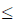1 is -∞ to 0. Hence, option 4.

IIFT Quantitative Ability MCQ Quiz - 1 - Question 13

Centre-point is a town named so because its centre lies on the origin when its country’s map is made using Cartesian co-ordinates. The only railway line in this town runs parallel to the y-axis at a distance of 4 km to the right of the centre of the town. The town planners now plan to build a highway perpendicular to the railway line. The road engineer has specified that the highway should satisfy (2x - y + 1) + k(x + 3y + 2) = 0, but has missed out on specifying k. What is the value of k?

Detailed Solution for IIFT Quantitative Ability MCQ Quiz - 1 - Question 13

Since the railway line runs parallel to the y-axis, its equation is of the form x = c.
Since it runs at a distance of 4 km to the right of the centre of the town, equation of the railway line is x = 4.
Equation o f the highway = (2x - y + 1) + k(x + 3y + 2) = 0. x(2 + k) + y(3k - 1) + 2k + 1 = 0 Since the highway is perpendicular to the railway line, the highway is parallel to the x-axis.

Hence, the co-efficient of x is 0.  Therefore 2 + k = 0; k = - 2 Hence, option 1.

IIFT Quantitative Ability MCQ Quiz - 1 - Question 14

Priya bought chocolates worth Rs. 358 for school kids. She gave each girl an almond flavored chocolate and every boy was given a coffee flavored chocolate. The cost of one coffee and one almond flavored chocolate was Rs. 4.80 and Rs. 3.70 respectively. What can be the number of chocolates of almond and coffee flavors that she bought?

Detailed Solution for IIFT Quantitative Ability MCQ Quiz - 1 - Question 14

Let x and y be the number of almond and coffee flavored chocolates.
She bought chocolates worth Rs. 358.

3.7x + 4.8y = 358 Since there is only one equation in two variables, the only way to solve it is by substituting answer options.
Only the values in option 2 satisfy this equation.
Hence, option 2.

IIFT Quantitative Ability MCQ Quiz - 1 - Question 15

Mr. Mickey went to buy a suit. The marked price of the suit was Rs. 2,000. The shopkeeper was ready to offer a 20% discount on the marked price. Also, Mr. Mickey had a voucher which he could use anytime in the next 12 months to avail a further discount of 25% on this selling price. When he was just about to purchase the suit, he saw that there was an upcoming sale in the next month which would guarantee him a reduction in the marked price by 30%. If the shopkeeper is ready to give him the same discount (i.e. 20%) on this reduced price during the sale, what is the percentage saving of Mr. Mickey if he buys the suit during the sale?

Detailed Solution for IIFT Quantitative Ability MCQ Quiz - 1 - Question 15

Without the sale, Mr. Mickey gets a 20% discount and then a 25% discount.
During the sale, the shopkeeper gives Mr. Mickey the 20% discount as well as the 25% voucher (which is also valid).
Mr. Mickey also gets a further reduction of 30% due to the sale.
Thus, the percentage saving of Mr. Mickey is nothing but the additional percentage discount that he gets apart from the 20% and 25% that he was already getting.
Since he gets a 30% reduction, that is his savings.
Hence, option 2.

Alternatively, It is given that the marked price of the suit is Rs. 2,000.
Mr. Mickey originally gets 20% off from the shopkeeper and a further discount of 25% on the reduced price due to his voucher.
Mr. Mickey's cost price = 0.75 x 0.8 x 2000 = Rs. 1,200.

During the sale, Mr. Mickey gets a 30% reduction in the marked price, then a 20% discount from the shopkeeper and finally a 25% discount due to the voucher.
Mr. Mickey's cost price = 0.7 x 0.75 x 0.8 x 2000 = Rs. 840.

The percentage saving of Mr. Mickey = [(1200 - 840)/1200)] x 100
= 30%

Hence, option 2.

IIFT Quantitative Ability MCQ Quiz - 1 - Question 16

Given the following system of equations:

[log5(2x + 5y)]2 - log5(2x + 5y)6 + 5 = 0 {where (2x + 5+) < 10}

4log2log3(3x +2y) - log3(3x +2y)+ 6 = 0 {where (3x + 2y) < 10}

What will be the value of 8x + 12y?

Detailed Solution for IIFT Quantitative Ability MCQ Quiz - 1 - Question 16

Let log5(2x + 5y) = Z

From the first given equation we get, Z2 - 6 Z + 5 = 0.
Z = 1 or 5 i.e. log5(2x + 5y) = 1 or 5 i.e. 2x + 5y = 5 or 5.

However, it is given that (2x + 5+) < 10.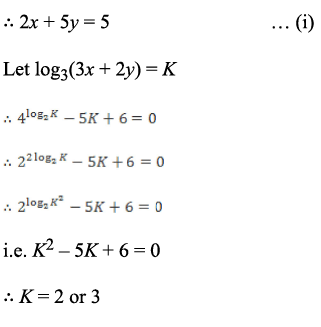i.e. log3(3x + 2y) = 2 or 3.

i.e. 3x + 2y = 9 or 27 However, it is given that (3x + 2y) < 10.

3x + 2y = 9 ... (ii)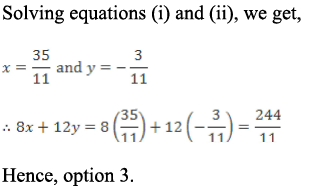IIFT Quantitative Ability MCQ Quiz - 1 - Question 17

What is the difference between the count of odd numbers between and including 300 and 500 and not divisible by 11, and the count of even numbers between and including 100 and 300 and not divisible by 5?

Detailed Solution for IIFT Quantitative Ability MCQ Quiz - 1 - Question 17

Odd numbers between 300 and 500 divisible by 11 are 319, 341, 363, 385, 407,429, 451,473 and 495.
There are 9 such numbers.
Count of odd numbers between 300 and 500 = 100 Count of odd numbers between 300 and 500 not divisible by 11 = 100- 9 = 91
Even numbers between 100 and 300 divisible by 5 are 100, 110, 120, 130, 140,..., 280, 290, 300
There are 21 such numbers.
Count of even numbers between 100 and 300 = 101

Count of even numbers between 100 and 300 not divisible by 5 = 101-21 = 80
Required difference = 91 - 80 =11 Hence, option 2.

IIFT Quantitative Ability MCQ Quiz - 1 - Question 18

A train takes 40 seconds to cross a cyclist travelling at a speed of 10 kmph in the opposite direction. Another train of same length and 50% faster speed takes 30 seconds to cross a man standing in a station. What is the length of the train?

Detailed Solution for IIFT Quantitative Ability MCQ Quiz - 1 - Question 18

Let the length of the trains be l.
Since the speed of 2nd train is 50% more, let the speeds of the 1st and 2nd train be 2x kmph and 3x kmph respectively.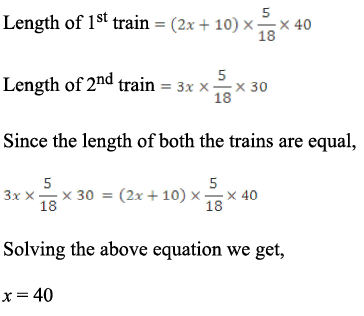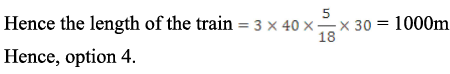IIFT Quantitative Ability MCQ Quiz - 1 - Question 19

There are a certain number of stones arranged along a straight line. Mohan picks up the first stone and puts it inside his bag. He breaks the second stone into 2 parts and puts them inside his bag. He breaks the third stone into 3 parts and puts them inside his bag and so on until the last stone. Later he realized that he forgot to break one more stone other than the first stone. Unable to recollect which stone it was, he went to a local mathematician for help. The mathematician counted the number of stones inside the bag and told him that he forgot to break the 17th stone. How many stones were there in the bag?

Detailed Solution for IIFT Quantitative Ability MCQ Quiz - 1 - Question 19

It has been shown that p( p + 1 ) = 2(N + 16). Both p and N are integers.
Now, the options represent potential values of N.
Use the values of N from the options and solve for p. See if you get integral value of p.
Option 1: N= 5033.

p(p + 1) = 2(5033 + 16) = 10098 Now, 100 x 101 = 10100

Thus, no integral value of p satisfies this equation.
Option 2: N = 20085

p(p+ 1) = 2(20085 + 16) = 40202 Now, 200 x 201 = 40200 Thus, no integral value ofp satisfies this equation.
Option 3: N = 80183.

p(p + 1) = 2(80183 + 16) = 160398 Now, 400 x 401 = 160400

Thus, no integral value of p satisfies this equation.
Hence, option 4.
Note: You can verify that N = 320384 gets satisfied for p = 800, but you need not calculate it in the exam as the other options have been eliminated.

IIFT Quantitative Ability MCQ Quiz - 1 - Question 20

A ball rebounds to 4/5th of the height it is dropped from. What is the total distance (in m) that the ball travels before coming to rest if it is dropped from 250 m?

Detailed Solution for IIFT Quantitative Ability MCQ Quiz - 1 - Question 20

Let s be the total distance by the ball before coming to rest.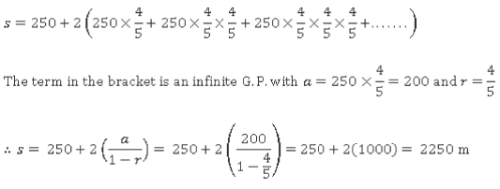Hence, option 3.

IIFT Quantitative Ability MCQ Quiz - 1 - Question 21

A survey was conducted by a firm that develops games to identify the games that kids like. It is perceived that 90 percent of the surveyed kids like GTA and 80 percent of the surveyed kids like FIFA. Further, it is believed that those who like GTA also like Assassin’s Creed but not FIFA, with a conditional probability as high as 0.90. Also, those who like GTA also like FIFA and not Assasin's Creed with a conditional probability of 0.067. There is no kid who likes only GTA. If a randomly surveyed kid likes GTA, what is the probability that he likes all three games?

Detailed Solution for IIFT Quantitative Ability MCQ Quiz - 1 - Question 21

Assume that 100 kids were surveyed.

Hence, 90% of these i.e. 90 kids like GTA.
Now, the randomly selected kid likes GTA. Hence, this kid can be chosen in 90C1 = 90 ways
Now, number of kids who like GTA and Assassin’s creed but not FIFA = 0.90 x 90 = 81. Similarly, number of kids who like GTA and FIFA but not Assasin's Creed = 0.067 x 90 ~ 6.

Since there is no kid who likes only GTA, the number of kids who like all three games is: 90 - (81 + 6) = 3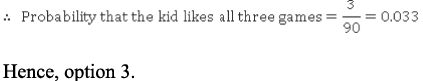IIFT Quantitative Ability MCQ Quiz - 1 - Question 22

There are 10 distinct consonants and 5 distinct vowels. How many words can be made using 6 consonants and 4 vowels such that no two vowels are adjacent to each other?

Detailed Solution for IIFT Quantitative Ability MCQ Quiz - 1 - Question 22

6 out of 10 consonants can be selected in 10C6 ways while 4 out of 5 vowels can be selected in 5C4 ways.
Let C1, C2, C3, C4, C5, and C6 be the 6 consonants. Since no two vowels are adjacent to each other, place the consonants first as shown below. _C1_C2_C 3_C 4_C 5_C 6_
Now, the 4 vowels can occupy any four places out of the seven blank spaces shown above. This can be done in 7C4 ways.

Since all the consonants as well as all the vowels are distinct, the consonants and vowels can be respectively arranged in 6! and 4! ways.
Total number of words = 10C6 x 5C4 x 7C4 x 6! x 4! = 10C6 x 5C4 x 7C4 x 17280
Hence, option 1.

## IIFT Mock Test Series

1 docs|46 tests
 Use Code STAYHOME200 and get INR 200 additional OFF Use Coupon Code
Information about IIFT Quantitative Ability MCQ Quiz - 1 Page
In this test you can find the Exam questions for IIFT Quantitative Ability MCQ Quiz - 1 solved & explained in the simplest way possible. Besides giving Questions and answers for IIFT Quantitative Ability MCQ Quiz - 1, EduRev gives you an ample number of Online tests for practice

## IIFT Mock Test Series

1 docs|46 tests

### How to Prepare for CAT

Read our guide to prepare for CAT which is created by Toppers & the best Teachers